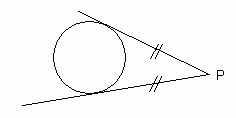SEARCH HOMEMath Central Quandaries & QueriesQuestion from Sean, a student: Four lines are tangent to a circle that form a quadrilateral.  It appears that the quadrilateral is a trapeziod but this is not a given.  Prove that the combined lengths of two opposing sides of the quadrilateral are equal to the combined lengths of the other two opposing sides of the quadrilateral.Hi Sean.One of the properties of circle geometry is that the distance from any external point P to a tangent point on a circle is equal to the distance to the other tangent point to P.

With your quadrilateral, you have four external points, so you can apply this concept four times and you will find your problem is solved.

Hope this helps,
Stephen La Rocque.Math Central is supported by the University of Regina and The Pacific Institute for the Mathematical Sciences.